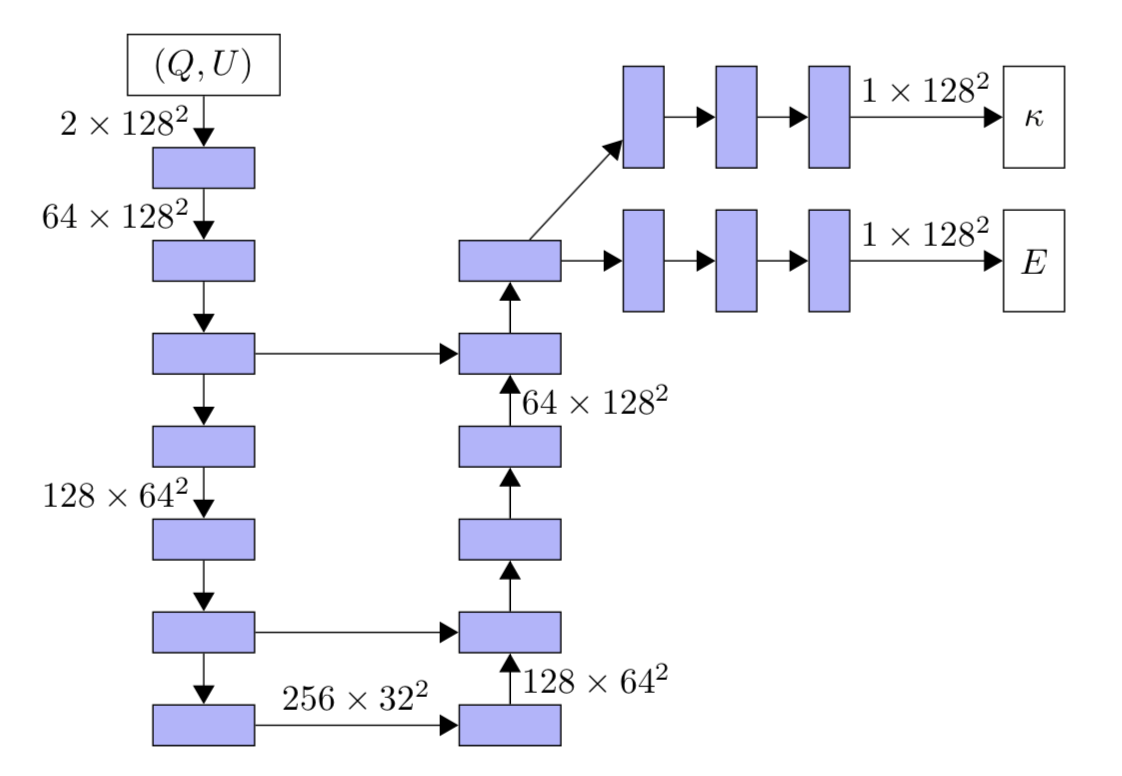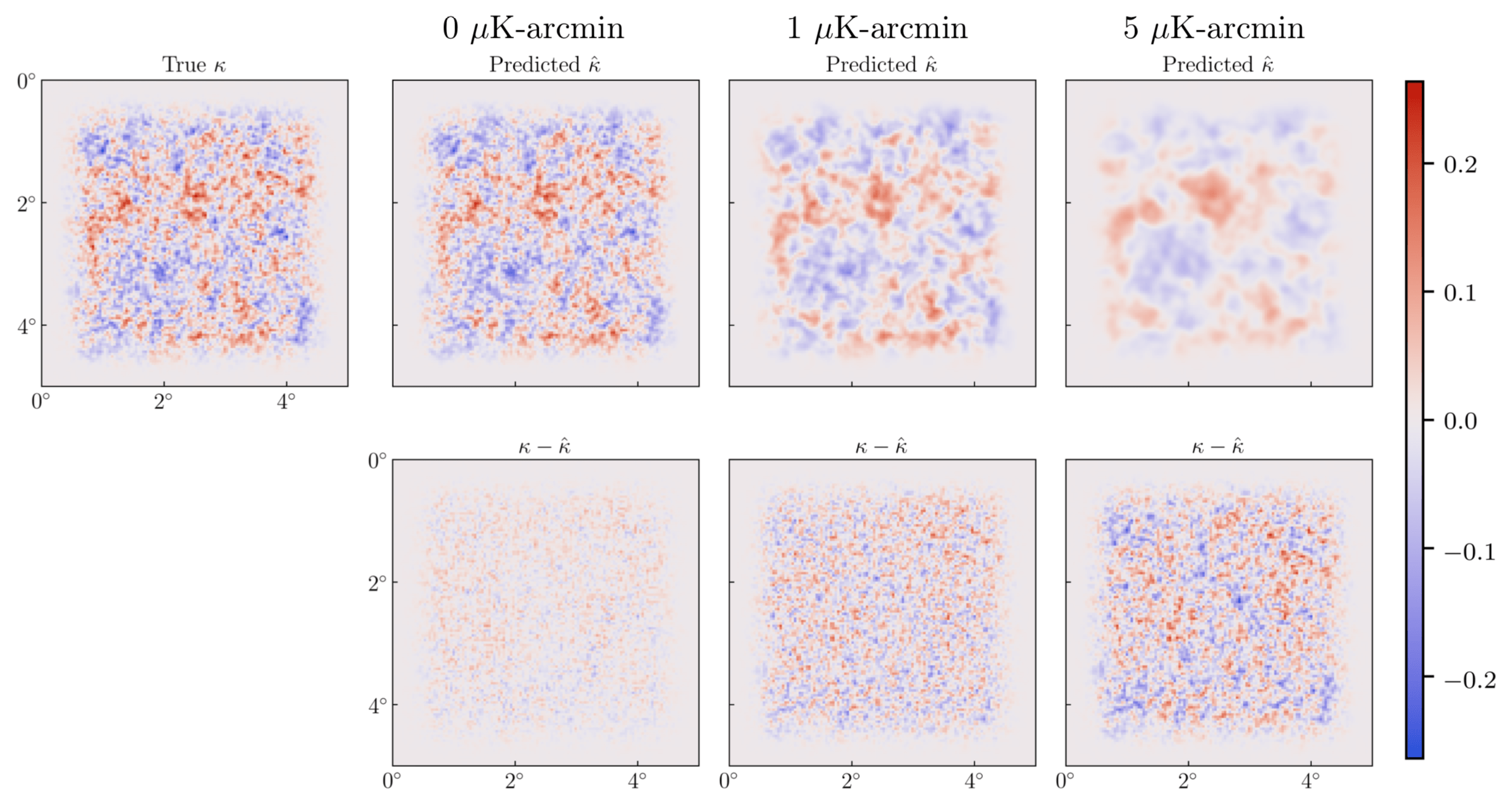# Learning to Warp the Earliest Cosmic Light

#### Deep CMB: Lensing Reconstruction of the Cosmic Microwave Background with Deep Neural Networks

###### By João Caldeira (jcaldeira@uchicago.edu); W. L. Kimmy Wu; Brian Nord; Camille Avestruz; Shubhendu Trivedi; Kyle T. Story

This work uses convolutional neural networks to reconstruct key features of the Cosmic Microwave Background (CMB) with an accuracy higher than current standard methods. Deep neural networks learn to measure the gravitational lensing signal from simulated maps of the Cosmic Microwave Background.

#### The Earliest LightFigure A – Temperature map of the Cosmic Microwave Background (CMB) from the Planck and WMAP experiments.

The Cosmic Microwave Background (CMB) is the earliest detectable light in our 13-billion-year-old Cosmos, emitted 400,000 years after the Big Bang. The CMB contains a treasure trove of information about the Universe: How old is it? What’s its shape? What’s it made of? While no earlier light can pierce this veil, we can use light from the CMB to infer earlier information. For example, the theory of Inflation predicts an imprint of primordial gravitational waves in the CMB.  We may one day detect these imprints… if we can disentangle them from similar-looking features.

#### Warped Perspective

One of the critical signals to disentangle from the CMB is gravitational lensing, a warping effect. Einstein’s General Relativity is our modern theory of gravity. John Wheeler summarizes it succinctly with the following: “Matter tells space how to curve, and space tells matter how to move.” Light follows a straight path in space, but when space is bent, so is the path of the light. In an astronomical context, as light from a distant object travels past matter on its way to us, the path of that light is bent, or warped.

Specifically, light from the distant CMB experiences gravitational lensing on its way to us. This ever-so-slightly distorts the image maps of the CMB, which lead to additional patterns in the map.  Each component that contributes to our observed CMB maps can be represented with a corresponding map.  E-mode and B-mode maps show two directions of polarized light that describe the patterns in the CMB, respectively the parallel/perpendicular and curlicue components.  The gravitational convergence map κ is a representation of the matter (between the CMB and the observer) that causes the lensing.Figure C – “An exaggerated example of the lensing effect on a 10 deg × 10 deg field. Top: (left-to-right) unlensed temperature field, unlensed E-polarization field, spherically symmetric deflection field d(n). Bottom: (left-to-right) lensed temperature field, lensed E-polarization field, lensed B-polarization field. The scale for the polarization and temperature fields differ by a factor of 10.” (Figure 1 of Hu and Okamoto, 2006 https://arxiv.org/abs/astro-ph/0111606)

#### Standard Lensing Reconstruction Methods

The Quadratic Estimator (QE) sets the current standard method for measuring the amount of matter that produces the observed amount of lensing. This method works analogously to a filter that can pick out distortions from a piece of music. If someone were to introduce a distortion to a song and then shift the high frequencies to low frequencies (and vice versa), one can use the QE to find how much shifting occurred.  In this analogy, the frequency shifting is like the lensing. There is also another method based on maximum likelihood estimation, which is less tested. We compare to an approximation of this method.

#### Neural Network Reconstruction of LensingFigure D – the architecture of the ResUNet used in our work (Figure 4). Blue boxes correspond to convolutional layers.

Some neural networks are used for classification or measurement (i.e., regression) — for example, convolutional neural networks can classify galaxies and strong gravitational lenses. These networks take an input layer of a single image of hundreds of pixels and an output layer of a single label (classification) or an output layer of a number of parameters that can be measured (regression). For example, we can label a galaxy as a spiral or measure the brightness and number of spiral arms in that galaxy.

Other networks, like autoencoders, input images and output images. Between the input and output layers, lies a “latent space” wherein the image information is partially contained. In this work, we use a similar network architecture called a ResUNet.

This architecture permits us to compare a set of input images with some known information into a set of output images with other known information. With it, we reconstruct the gravitational lensing signal in simulated CMB data.Figure E – “We train neural networks to learn a mapping from the lensed (Q,U) maps into the unlensed E map and the gravitational convergence map κ, extracting the underlying fields from the observed quantities. Here we illustrate this mapping using one of the realizations in the training set. The maps correspond to a patch of the sky five degrees across.” (Figure 1 of our work.)

#### Results: Comparing ResUNets and Standard Methods

We compare predictions from standard methods and ResUNets for both E-mode maps and gravitational convergence κ maps.  We examine both the predicted map images and their Fourier Transforms, represented by power spectra. In Figure F, we show the difference between input maps and neural network-predicted maps at multiple noise levels.Figure F – “Example of gravitational convergence κ maps for the realization corresponding to the (Q,U) maps shown in Fig. 5. The true map (κ) is shown on the left. The ResUnet predictions of κˆ (top) and the related residuals κ − κˆ (bottom) are shown with increasing levels of noise (0, 1, 5μK-arcmin; left to right). Without noise, κ recovery is better than E recovery, and this is reflected here by the lack of large-scale structure in the left-most residual map. However, κ recovery suffers much more from the addition of noise to the inputs than E recovery, and once we reach 5 μK-arcmin only large-scale structure is visible in the predicted map.” (Figure 7 of our work)

We quantify the comparison between methods by evaluating the noise power spectra of maps predicted by each method. This allows us to compare (in a way that is standard in CMB analyses) how much of the true map is correctly estimated.  To high values of L, ResUNet has lower noise power than QE.  See the results in Figure G below.Figure G – “To further evaluate the quality of κ reconstruction by ResUNets at different input noise levels, we compare the noise spectra from ResUNets to those from quadratic estimators. We see that the results have 50 − 70% less noise than quadratic estimator reconstructions across a wide range of angular scales L. For input noise of 5 μK-arcmin, performance quickly degrades for L > 2000.” (Figure 9a in our work)

We also perform a null test to check that the network returns null results when we feed input maps with no lensing in them: the recovery is accurate to percent-level. Furthermore, we perform tests to assess the sensitivity of the network to cosmological parameters of the training data: the network is sensitive.

#### Outlook

In this work, we show that ResUNets outperform the QE by 50 − 70% across a wide range of angular scales. We perform a number of tests to demonstrate this proof of concept. We also perform comparisons in a context that is natural for the astrophysical probe at hand: we establish an uncertainty measure via the noise power spectrum. It is critical that the community develops clear metrics for comparison that are interpretable in astronomical contexts. In the future, we look toward recovering other signals, like the primordial B modes and dust, as well as to predicting cosmology.